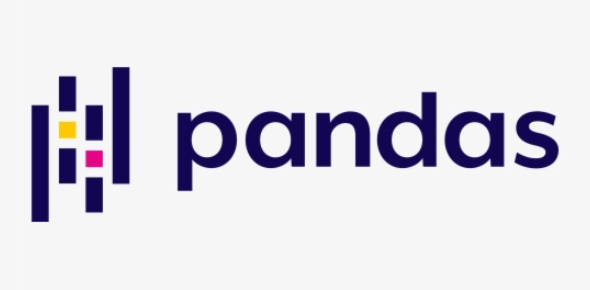# Programming Quiz: Pandas Software Library (True/False)

10 Questions | Total Attempts: 61SettingsAre you well familiar with the Pandas software library? It was written for Python programming language, with the ability to provide data structures and operations for data manipulation and analysis. So, if you know Pandas well, then can you answer these questions as well?

• 1.
Series is a one-dimensional labeled array capable of holding any data type.
• A.

True

• B.

False

• 2.
If data is an ndarray, index must be the same length as data.
• A.

True

• B.

False

• 3.
Panel is a container for Series, and DataFrame is a container for dataFrame objects.
• A.

True

• B.

False

• 4.
Pandas consist of static and moving window linear and panel regression.
• A.

True

• B.

False

• 5.
The iteritems() brings horizontal subsets from a dataframe
• A.

True

• B.

False

• 6.
Sorting refers to arranging values in a particular order.
• A.

True

• B.

False

• 7.
To access subset of a dataframe we can use loc() method.
• A.

True

• B.

False

• 8.
The value NaN/NAT/None are the same in Pandas.
• A.

True

• B.

False

• 9.
When you create a dataframe object, it gets its row numbers and column labels automatically.
• A.

True

• B.

False

• 10.
Scatter_matrix is the name of Pandas library tools used to create a scatter plot matrix
• A.

True

• B.

False

Related TopicsBack to top